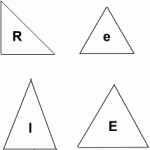A tetrahedron (plural tetrahedra) is a solid with four triangular faces. How many different tetrahedra can you make using the four different types of triangle shown in the diagram if you have an unlimited number of each type?

Type R are right angled isosceles triangles with sides a, a and b units.
Type E are large equilateral triangles with sides of length b units.
Type e are small equilateral triangles with sides of length a units.
Type I are isosceles triangles with sides of length a, b and b units.

How can you be sure you have found them all?